Select Page

# Transition Function in PDA

There are three basic cases in Pushdown automata (PDA) to well understand the functionality of transition function.

As we Know that transition function is Q × (∑ ∪ {ε}) x Γ  à Q × Γ *. Where

• Γ represents the symbol which is at the Top of Stack before transition function
• Γ* represents the all symbols which are present in Stack after Transition function.

## Example of PDA

Design a PDA for accepting a language {0n1n | n>=1}.

According to given language, consider an Input string which has equal number of 0’s and 1’s, as “0011∈”. Whenever the epsilon (∈) occurs as input, then that transition is the last transition.

So, we will apply a very simple logic is that for every single ‘1’ only one ‘0’ should get POP out from the stack.

## Explanation of Transition Function

Let understand all three cases of transition function with given input string

Case 01: If the symbols of Γ and Γ* are different then PUSH the symbol of Γ in stack.

To perform the PUSH Operation for the first input “0” the transition function and detail diagram are given below

δ(q0, 0, Z0) = (q0, 0Z0)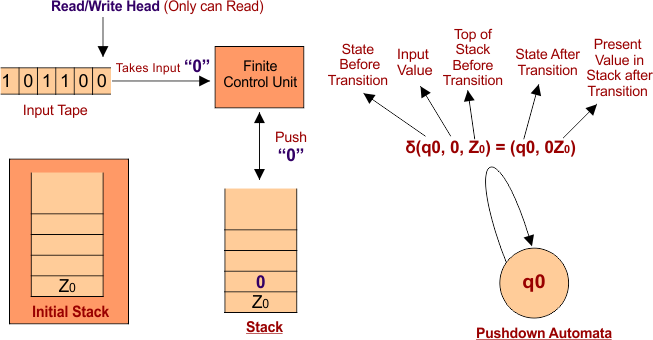Note: Z0 is stack default value which is also known as epsilon.

To perform the PUSH Operation for the second input “0” the transition function and detail diagram are given below

δ (q0, 0, 0) = (q0, 00Z0)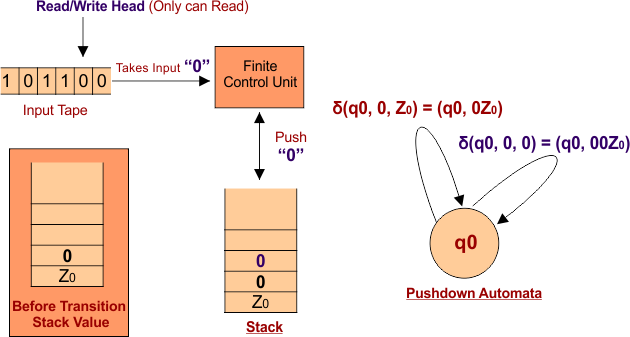Note: After each transition the state can either change or remain the same.

Case 02: If the value of Γ is non-empty but the value of Γ* is epsilon (empty) then POP the value of Γ from stack. Let see the following transition function example

To perform the POP Operation for the third input “1” the transition function and detail diagram are given below

δ(q0, 1, 0) = (q0, ∈)  // Now stack hold value “0Z0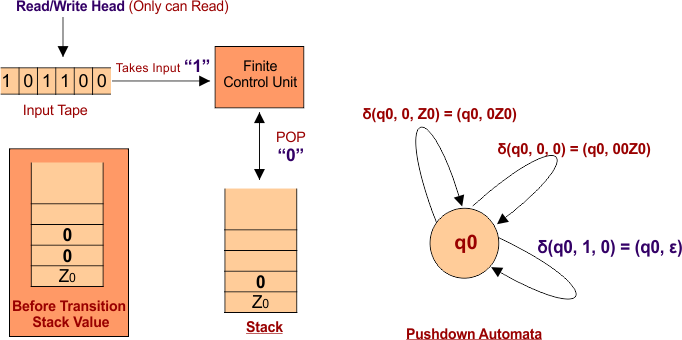To perform the POP Operation for the fourth input “1” the transition function and detail diagram are given below

δ(q0, 1, 0) = (q0, ∈)  // Now stack hold value “Z0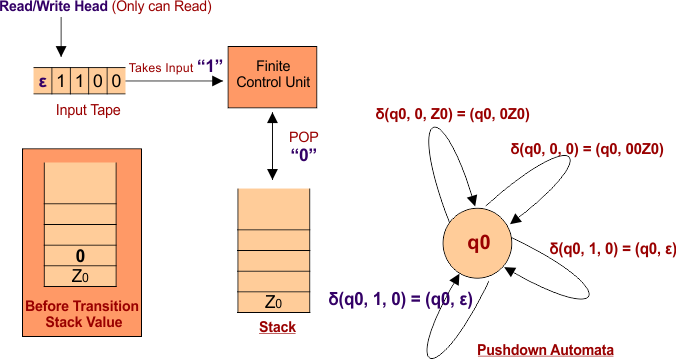Note: It means both values are POP out from stack through above transition functions.

Case 03: If the value of Γ and Γ* are both the same (i.e. both empty) in value then there will be no change in stack. Let see the following transition function example

As at this stage, the stack is empty (Z0) and last Input string having last symbol is epsilon (∈). So, there will no change in stack. The transition function with detail diagram is given below

δ (q0, ∈, Z0) = (q1, ∈)   // Z0 represent epsilon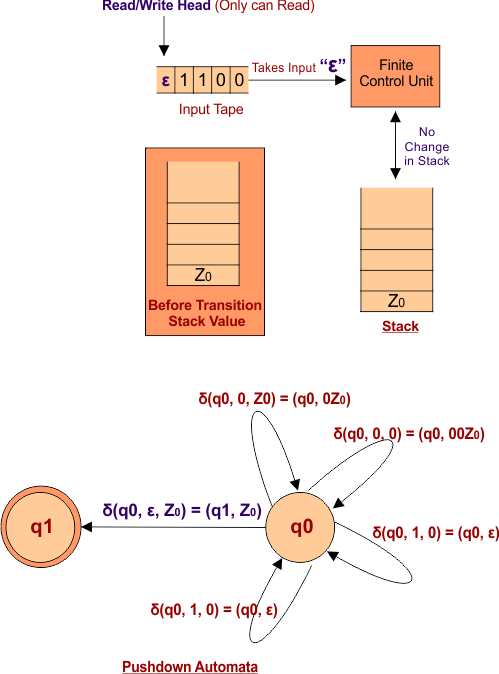Above transition function has no effect on stack but can change the current state (i.e. q0 to q1).

 In Pushdown Automata (PDA), Only One Input symbol is read at a time.  Only one input symbol is either PUSH or POP in stack at a time.

Help Other’s By Sharing…